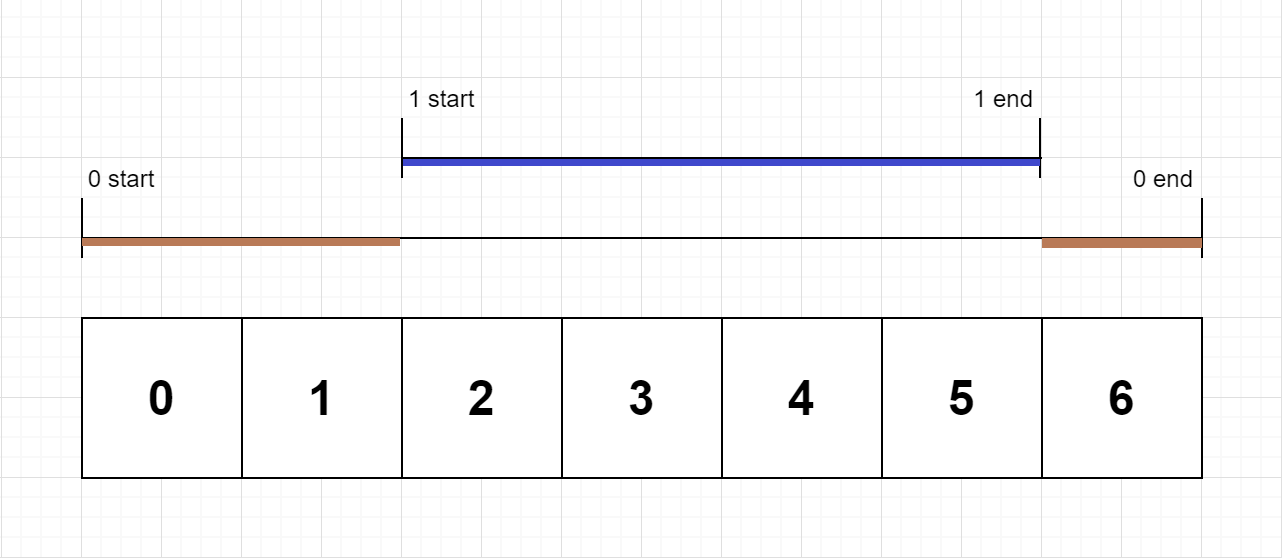# GeetCode Hub

On a single-threaded CPU, we execute a program containing `n` functions. Each function has a unique ID between `0` and `n-1`.

Function calls are stored in a call stack: when a function call starts, its ID is pushed onto the stack, and when a function call ends, its ID is popped off the stack. The function whose ID is at the top of the stack is the current function being executed. Each time a function starts or ends, we write a log with the ID, whether it started or ended, and the timestamp.

You are given a list `logs`, where `logs[i]` represents the `ith` log message formatted as a string `"{function_id}:{"start" | "end"}:{timestamp}"`. For example, `"0:start:3"` means a function call with function ID `0` started at the beginning of timestamp `3`, and `"1:end:2"` means a function call with function ID `1` ended at the end of timestamp `2`. Note that a function can be called multiple times, possibly recursively.

A function's exclusive time is the sum of execution times for all function calls in the program. For example, if a function is called twice, one call executing for `2` time units and another call executing for `1` time unit, the exclusive time is `2 + 1 = 3`.

Return the exclusive time of each function in an array, where the value at the `ith` index represents the exclusive time for the function with ID `i`.

Example 1:```Input: n = 2, logs = ["0:start:0","1:start:2","1:end:5","0:end:6"]
Output: [3,4]
Explanation:
Function 0 starts at the beginning of time 0, then it executes 2 for units of time and reaches the end of time 1.
Function 1 starts at the beginning of time 2, executes for 4 units of time, and ends at the end of time 5.
Function 0 resumes execution at the beginning of time 6 and executes for 1 unit of time.
So function 0 spends 2 + 1 = 3 units of total time executing, and function 1 spends 4 units of total time executing.
```

Example 2:

```Input: n = 1, logs = ["0:start:0","0:start:2","0:end:5","0:start:6","0:end:6","0:end:7"]
Output: 
Explanation:
Function 0 starts at the beginning of time 0, executes for 2 units of time, and recursively calls itself.
Function 0 (recursive call) starts at the beginning of time 2 and executes for 4 units of time.
Function 0 (initial call) resumes execution then immediately calls itself again.
Function 0 (2nd recursive call) starts at the beginning of time 6 and executes for 1 unit of time.
Function 0 (initial call) resumes execution at the beginning of time 7 and executes for 1 unit of time.
So function 0 spends 2 + 4 + 1 + 1 = 8 units of total time executing.
```

Example 3:

```Input: n = 2, logs = ["0:start:0","0:start:2","0:end:5","1:start:6","1:end:6","0:end:7"]
Output: [7,1]
Explanation:
Function 0 starts at the beginning of time 0, executes for 2 units of time, and recursively calls itself.
Function 0 (recursive call) starts at the beginning of time 2 and executes for 4 units of time.
Function 0 (initial call) resumes execution then immediately calls function 1.
Function 1 starts at the beginning of time 6, executes 1 units of time, and ends at the end of time 6.
Function 0 resumes execution at the beginning of time 6 and executes for 2 units of time.
So function 0 spends 2 + 4 + 1 = 7 units of total time executing, and function 1 spends 1 unit of total time executing.
```

Example 4:

```Input: n = 2, logs = ["0:start:0","0:start:2","0:end:5","1:start:7","1:end:7","0:end:8"]
Output: [8,1]
```

Example 5:

```Input: n = 1, logs = ["0:start:0","0:end:0"]
Output: 
```

Constraints:

• `1 <= n <= 100`
• `1 <= logs.length <= 500`
• `0 <= function_id < n`
• `0 <= timestamp <= 109`
• No two start events will happen at the same timestamp.
• No two end events will happen at the same timestamp.
• Each function has an `"end"` log for each `"start"` log.

class Solution { public int[] exclusiveTime(int n, List<String> logs) { } }